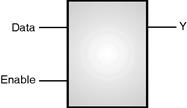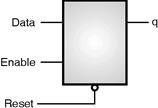Home > VHDL > Flipflops > D latch

1) D-Latch with Data and Enable :Enable Data y y + 1(next) 0 x 0 0 0 x 1 1 1 0 x 0 1 1 x 1

Following code shows the VHDL implementation of D latch with enable. If enable is 1, then y is equal to the data value.

library IEEE;

use IEEE.std_logic_1164.all;

entity d_latch is

port (enable, data: in std_logic;

y : out std_logic);

end d_latch;

architecture behave of d_latch is

begin

process (enable, data)

begin

if (enable = '1') then

y <= data;

end if;

end process;

end behave;

Level sensitive latch inferencing occurs when a signal is not assigned a value under all possible conditions within a process statement. If a signal is assigned a value in a least one condition and not assigned a value in at least one other condition, the signal needs to store its current value to be used in the next activation of the process.

library IEEE;

use IEEE.std_logic_1164.all;

entity d_latch is

port (a, b, Clear, Enable : in std_logic;

q : out std_logic);

END d_latch;

architecture rtl of d_latch is

begin

process (a, b, Clear, Enable)

begin

if (Clear = '1') then

q <= b;

elsif (Enable = '1') then

q <= a;

end if;

end process;

end rtl;

The following examples infer a D-latch with an asynchronous reset.library IEEE;

use IEEE.std_logic_1164.all;

entity d_latch_rst is

port (enable, data, reset: in std_logic;

q :out std_logic);

end d_latch_rst;

architecture behav of d_latch_rst is

begin

process (enable, data, reset)

begin

if (reset = '0') then

q <= '0';

elsif (enable = '1') then

q <= data;

end if;

end process;

end behav;

library ieee;

use ieee.std_logic_1164.all;

entity d_latch is

port (clk: in std_logic;

enable, din: in std_logic;

set, reset: in std_logic;

dout : out std_logic);

end d_latch;

architecture behave of d_latch is

begin

process (clk)

begin

if (enable = '1') then

if (clk'event and clk = '1') then

if set = '1' then

dout <= '1';

elsif reset = '1' then

dout <= '0';

else

dout <= din;

end if;

end if;

end if;

end process;

end behave;